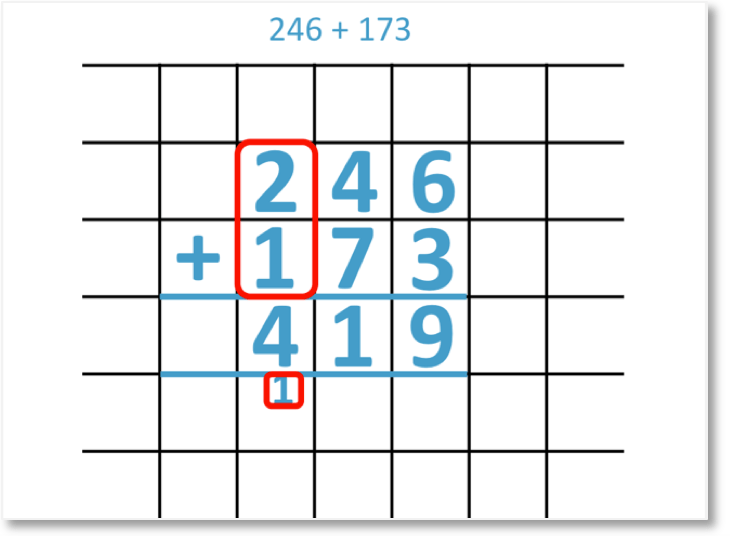• A digit is a one of the numerals: 0, 1, 2, 3, 4, 5, 6, 7, 8, or 9 that are used to make up a number.
• We can partition the digits in this addition into their hundreds, tens and units (ones) columns.
• We add the units, tens and hundreds of these numbers separately, starting from the column on the right.
• 2 + 5 = 7. There are 7 units (ones) in total.
• 5 + 1 = 6. There are 6 groups of ten in total.
• 3 + 2 = 5. There are 5 groups of one hundred in total.
• 352 + 215 = 567

We partition the digits into their hundreds, tens and units columns and add them separately from right to left.• 352 + 215 is set out with the first number above the second number.
• With 1 digit per box, each digit within the numbers are set out above each other.
• The units digit on the right, ‘2’ and ‘5’ are above each other.
• The digits in the tens and hundreds columns are also set out above each other.
• We start on the right in the units column and add the digits together

• 2 + 5 = 7.
• We move on to add the digits in the tens column.
• 5 + 1 = 6.
• Finally we add the digits in the hundreds column.
• 3 + 2 = 5.
• We can read the total as 567.Supporting Lessons#### 3-Digit Addition Without Regrouping: Interactive Questions

3-Digit Addition Without Regrouping: Interactive Questions

# How to Add Numbers Without Regrouping

## What is Addition Without Regrouping

Addition with regrouping is when one of the place value columns add up to a number that is 10 or greater. Only the ones digit is written down and the tens digit of the answer is regrouped to be added to the next column.

Addition without regrouping is when the digits add up to a number that is 9 or less. The answer can simply be written below each place value column. There is no carrying of tens or hundreds.

When talking about adding numbers, regrouping means the same as carrying. Carrying was originally a more commonly used word that applies only to adding numbers, however, the phrase regrouping is becoming more popular. Both words mean the same thing when performing addition.

In subtraction, regrouping means the same as borrowing.

## How to Add without Regrouping

1. Write the numbers directly above each other, lining up the place value columns.
2. Add the digits in each column, starting on the right and moving left.
3. Write the answer to each addition in the answer space directly below each column of digits.

For example, here is 352 + 215.

When teaching written addition methods, square grid paper is useful to ensure that the digits are lined up above each other.

The rule for addition without regrouping is to only write one digit in each position on the grid. Never write two digits in one place.

Step 1 is to write the numbers directly above each other.

Step 2 is to add the digits in each column.

We start on the right in the units column.2 + 5 = 7

We now add the digits in the tens column.5 + 1 = 6

We now move left to add the digits in the hundreds column.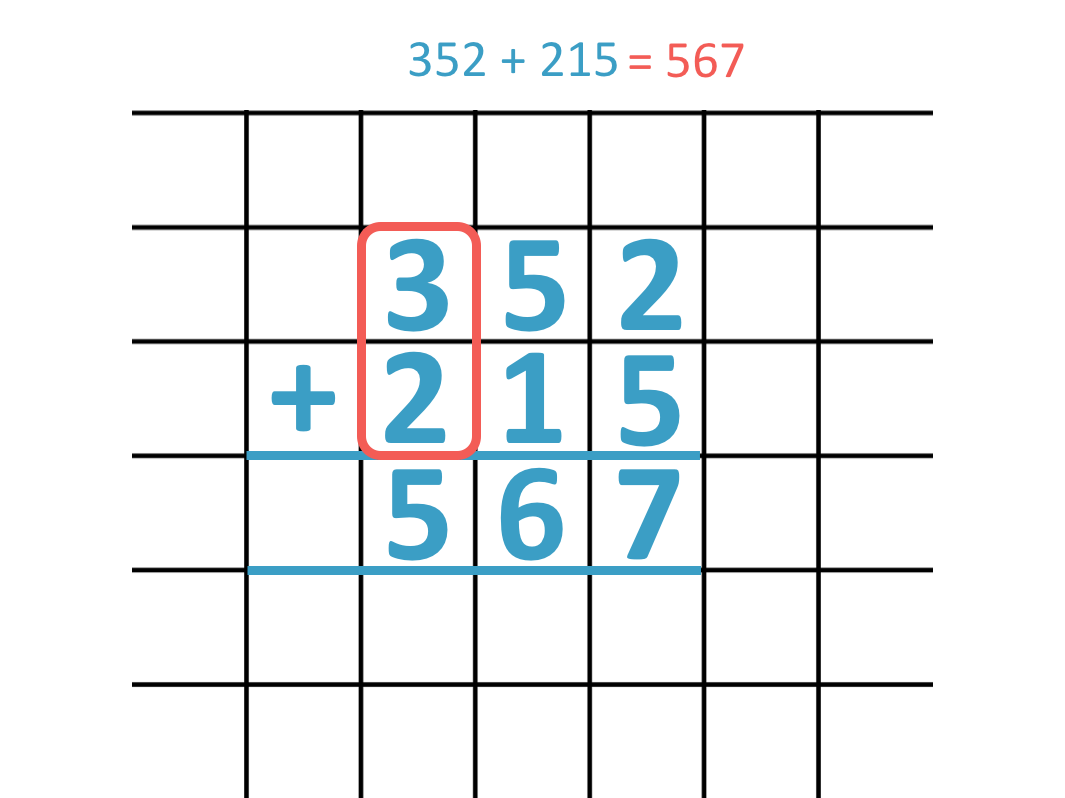3 + 2 = 5

We have now added the total number of ones, tens and hundreds by adding each digit separately.

352 + 215 = 567.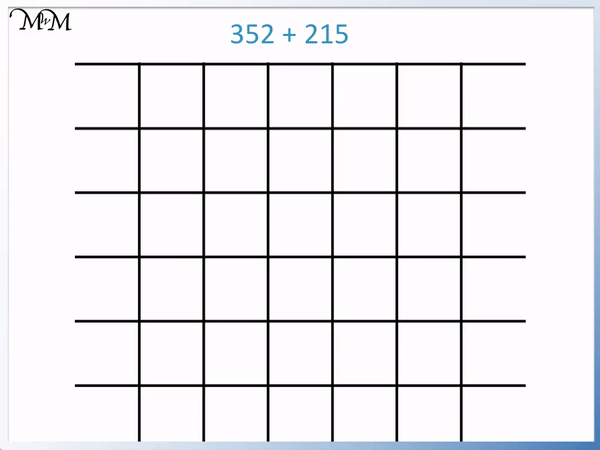We were able to add all digits without arriving at an answer larger than 9 for any digit. We did not have to use the regrouping process.

Regrouping is not always used. Regrouping is only used when the sum of digits in a place value column is ten or more. This is because we cannot write two digits in one column and we need groups of ten for the regrouping process.

When first teaching written addition, it is recommended to begin by looking at problems without regrouping, such as in this lesson.

Here is another example of adding two three-digit numbers using the column addition method.

Again, this example will not involve regrouping because when we add each digit we will not get an answer that is bigger than 9.

We have the three-digit addition of 531 + 248.

We line up the digits that are in the same place value columns above each other and then start adding the digits starting from the right.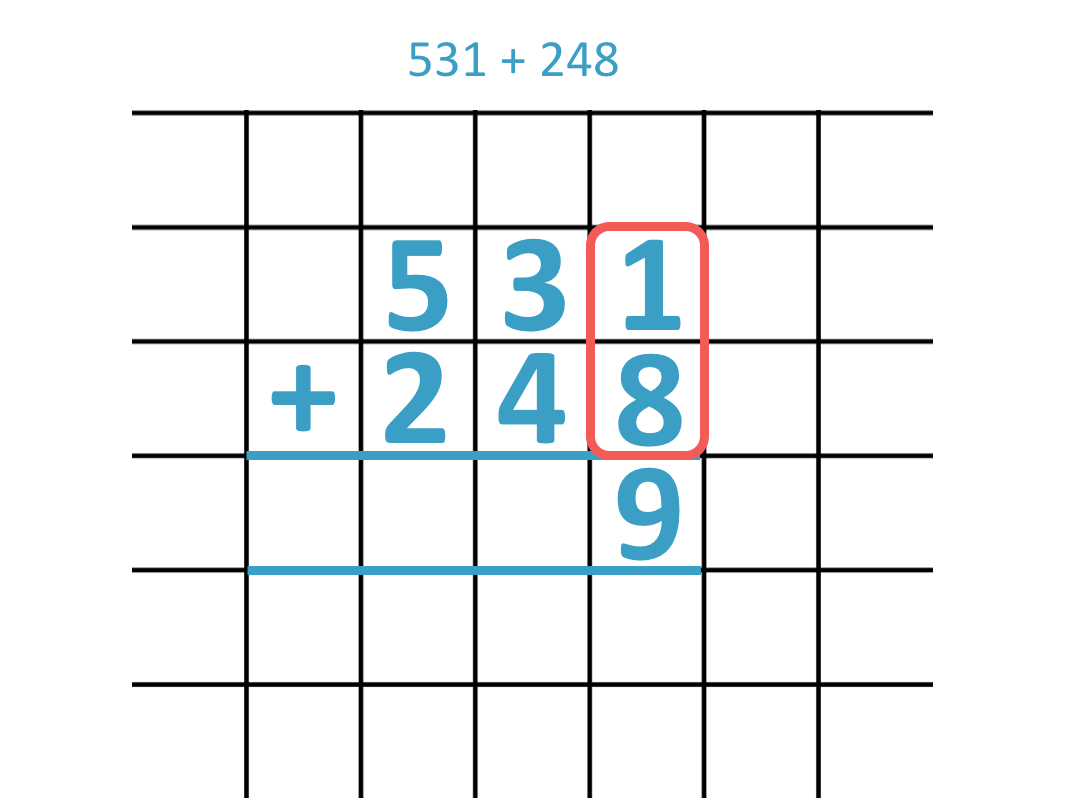Adding the digits in the ones column we have:

1 + 8 = 9

Now we add the digits in the tens column.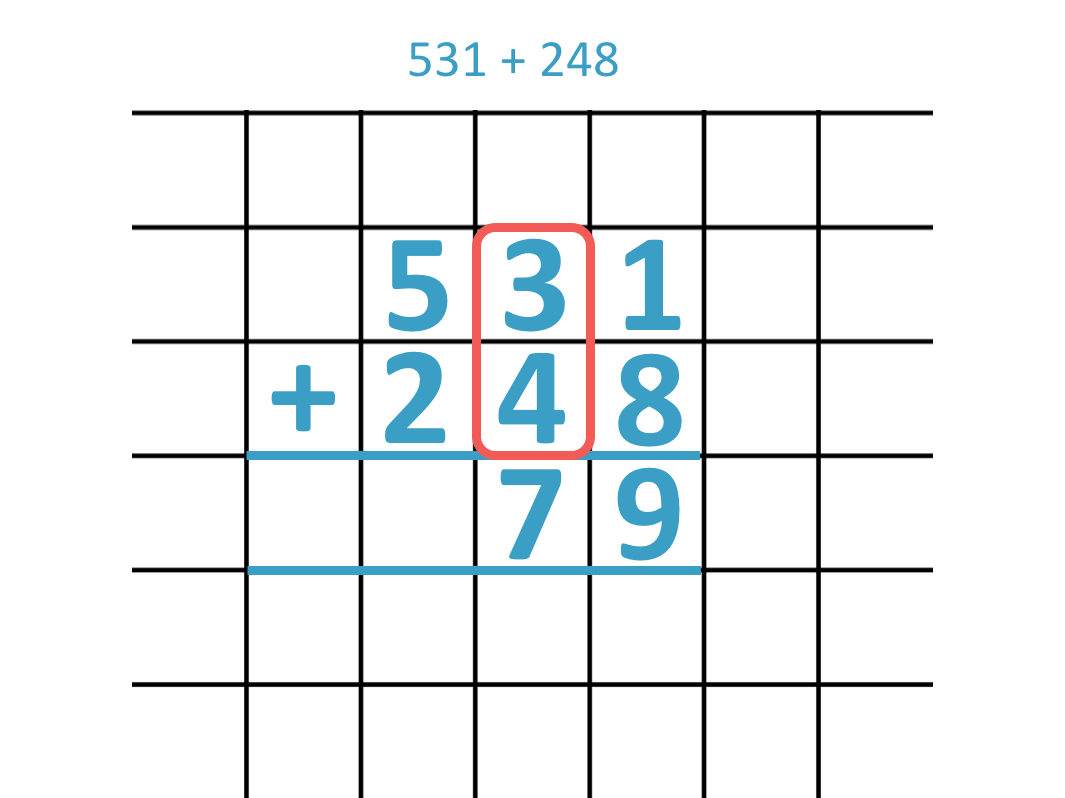3 + 4 = 7

Finally we move left onto adding the digits in the hundreds column.5 + 2 = 7

531 + 248 = 779

Here is the full written addition method, adding the two three digit numbers.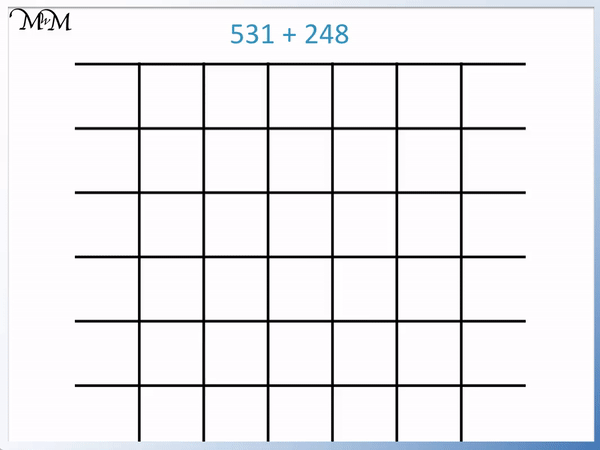Here is an example of addition without regrouping. 514 + 372 = 886. We can simply add the digits in each place value column.

We have 514 + 372.

We set out the column addition written method with the units, tens and hundreds digits written above each other.

We will add the digits separately from right to left, starting in the ones column.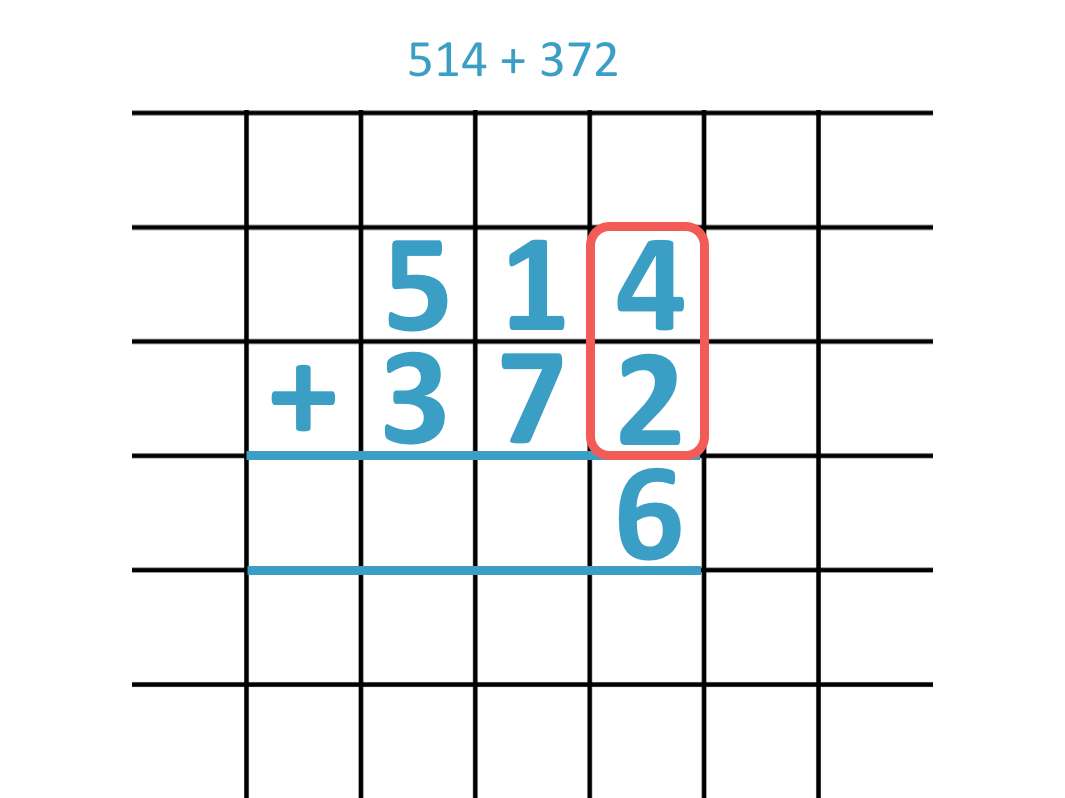4 + 2 = 6

We will now add the tens column digits.1 + 7 = 8

Now we will add the hundreds digits.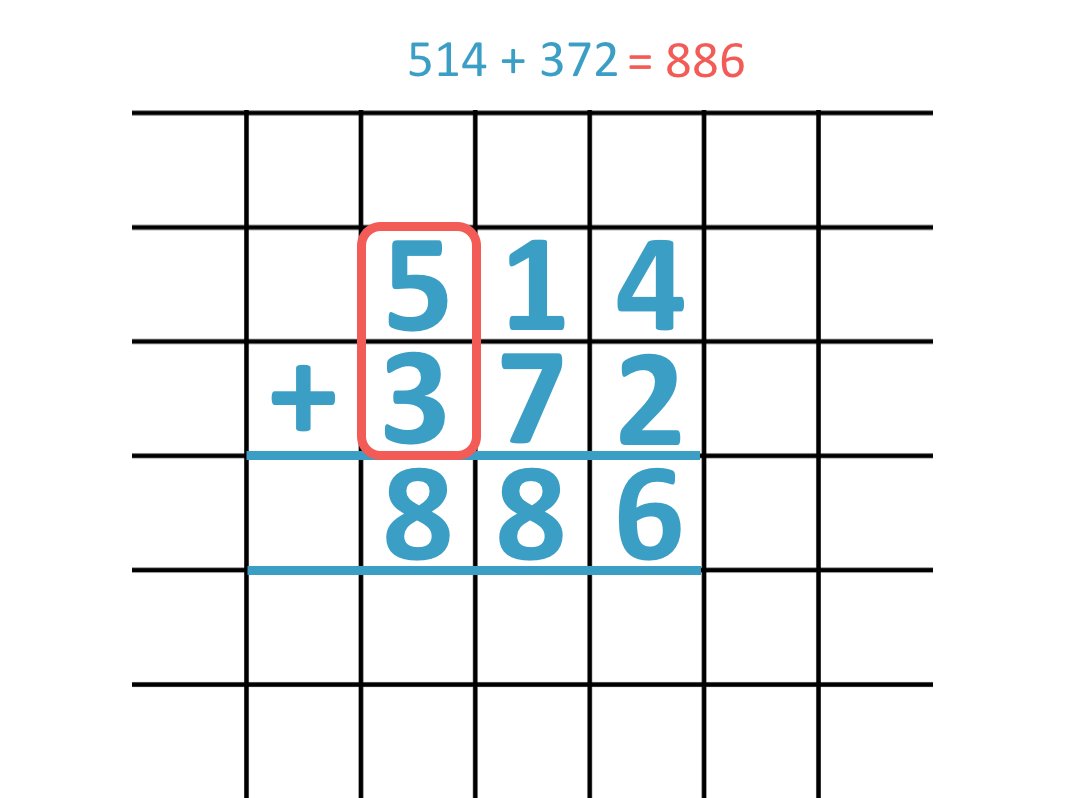We can see the full written addition method below.514 + 372 = 886

Don’t forget to download our PDF worksheets above for more practice of adding three-digit numbers without regrouping.

## How to Teach Addition Without Regrouping

Addition without regrouping can be taught using base ten blocks and arranging them into place value columns. The total number of each type of block in each column will represent the digits of the answer.

A digit is one of the numerals: 0, 1, 2, 3, 4, 5, 6, 7, 8 or 9 that we can combine together to write numbers.

To add numbers, line up each digit in each number above each other in place value columns. Then add each digit separately from right to left.

In this lesson, we are adding numbers without regrouping. This means that when we add the digits in each place value column together, we will not get an answer in each place value column that is larger than 9.

Here is our first example of adding two three-digit numbers.

We have 352 + 215.

We can partition the numbers into their hundreds, tens and units columns.352 is made up of 3 groups of one hundred, 5 groups of ten and 2 ones.

215 is made up of 2 groups of one hundred, 1 group of ten and 5 ones.When teaching column addition it can be helpful to first show the size of the numbers with counters or dienes blocks as in this example. It can help to remind us of the size of the number that each digit represents.

We now add the ones, tens and hundreds separately.

We start with the rightmost column, which is the units (or ones) column.

2 + 5 = 7We now add the groups of counters in the tens column.

5 + 1 = 6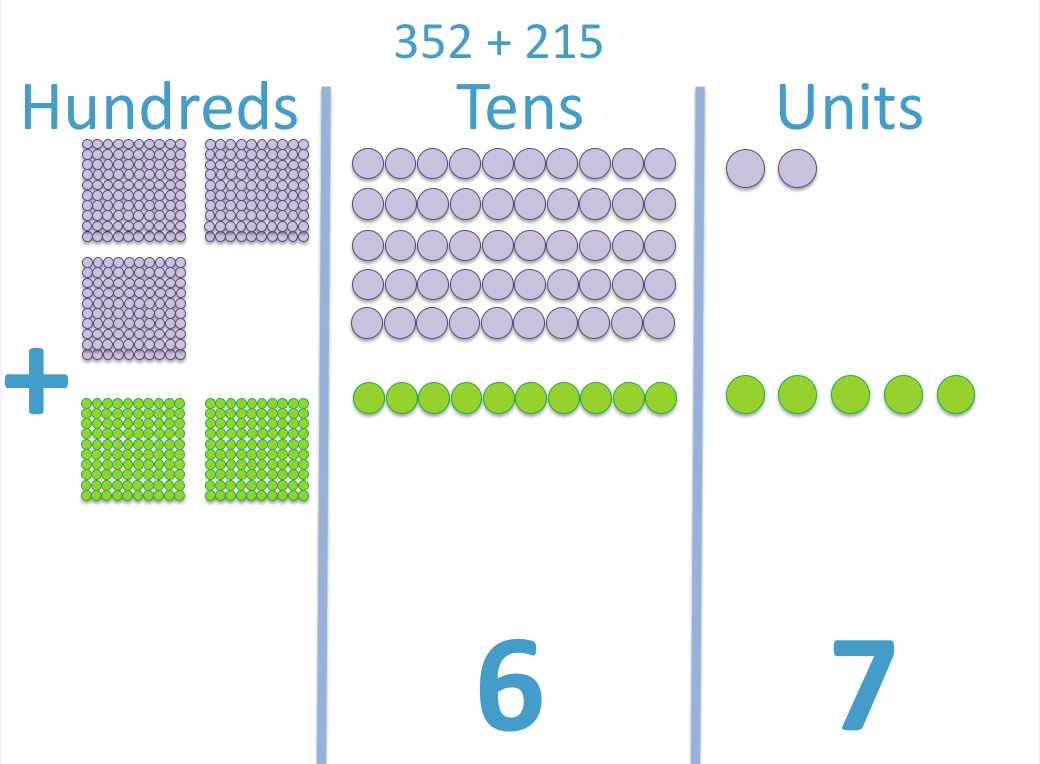We now add the groups of hundreds in the hundreds column.

3 + 2 = 5When teaching addition, it can be useful to start with physical objects and simply count them. Once the idea is undertood, it is helpful to move on to a written method and regularly practise following the procedure through and setting it out with one digit per box.

Particular focus should be given to lining up the digits in the same place value columns.